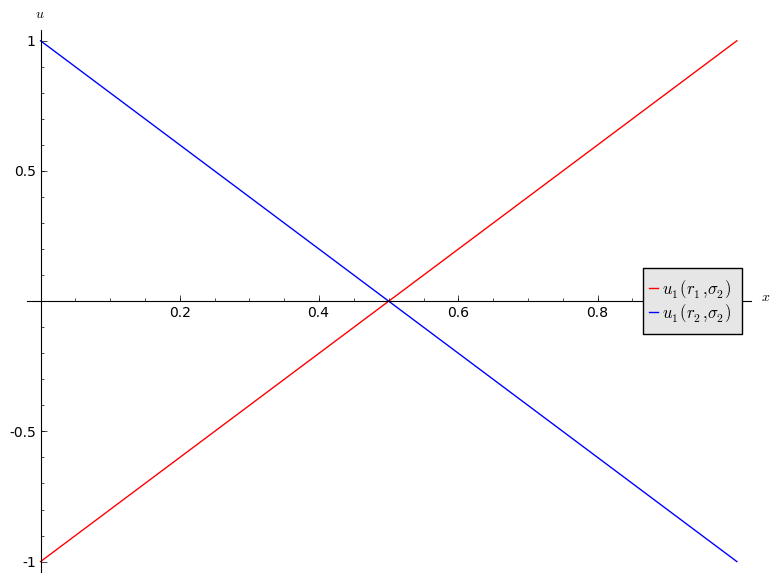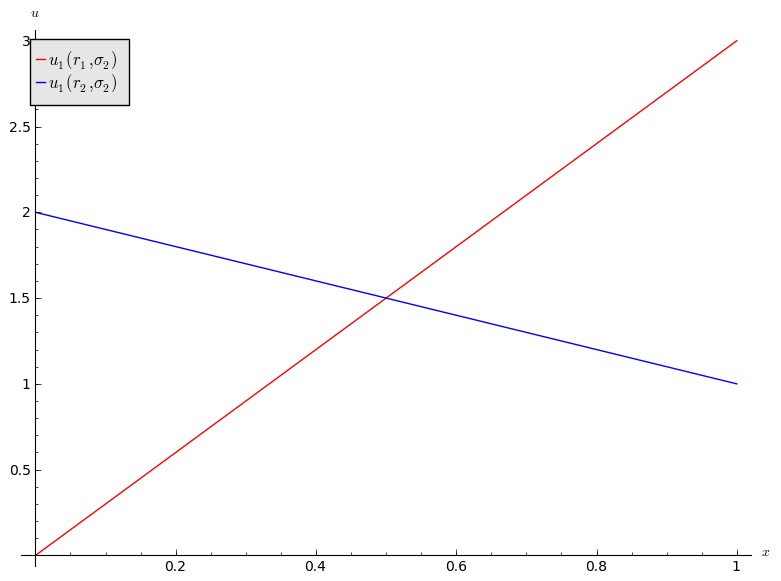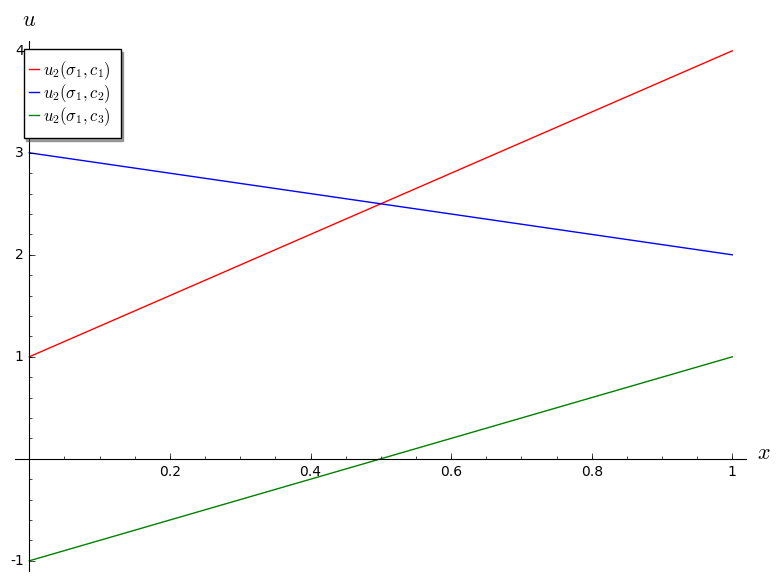## Recap

In the previous chapter we discussed:

• Predicting rational behaviour using dominated strategies;
• The CKR;

We did discover certain games that did not have any dominated strategies.

## Best response functions

### Definition of a best response

In an $$N$$ player normal form game. A strategy $$s^*$$ for player $$i$$ is a best response to some strategy profile $$s_{-i}$$ if and only if $$u_i(s^*,s_{-i})\geq u_{i}(s,s_{-i})$$ for all $$s\in S_i$$.

We can now start to predict rational outcomes in pure strategies by identifying all best responses to a strategy.

We will underline the best responses for each strategy giving ($$r_i$$ is underlined if it is a best response to $$s_j$$ and vice versa):

We see that $$(r_3,c_3)$$ represented a pair of best responses. What can we say about the long term behaviour of this game?

## Best responses against mixed strategies

We can identify best responses against mixed strategies. Let us take a look at the matching pennies game:

If we assume that player 2 plays a mixed strategy $$\sigma_2=(x,1-x)$$ we have:

andWe see that:

1. If $$x<1/2$$ then $$r_2$$ is a best response for player 1.
2. If $$x>1/2$$ then $$r_1$$ is a best response for player 1.
3. If $$x=1/2$$ then player 1 is indifferent.

Let us repeat this exercise for the battle of the sexes game.

If we assume that player 2 plays a mixed strategy $$\sigma_2=(x,1-x)$$ we have:

andWe see that:

1. If $$x<1/2$$ then $$r_2$$ is a best response for player 1.
2. If $$x>1/2$$ then $$r_1$$ is a best response for player 1.
3. If $$x=1/2$$ then player 1 is indifferent.

## Connection between best responses and dominance

### Definition of the undominated strategy set

In an $$N$$ player normal form game, let us define the undominated strategy set $$UD_i$$:

If we consider the following game:

We have:

$UD_1=\{r_1,r_2\}$ $UD_2=\{c_1,c_2\}$

### Definition of the best responses strategy set

In an $$N$$ player normal form game, let us define the best responses strategy set $$B_i$$:

In other words $$B_i$$ is the set of functions that are best responses to some strategy profile in $$S_{-i}$$.

Let us try to identify $$B_2$$ for the above game. Let us assume that player 1 plays $$\sigma_1=(x,1-x)$$. This gives:

Plotted:We see that $$c_3$$ is never a best response for player 2:

We will now attempt to identify $$B_1$$ for the above game. Let us assume that player two plays $$\sigma_2=(x,y,1-x-y)$$. This gives:

$u_1(r_1,\sigma_2)=xu_1(r_1,c_1)+yu_1(r_1,c_2)+(1-x-y)u_1(r_1,c_3)=3x+7y+5-5y-5x$ $u_1(r_2,\sigma_2)=xu_1(r_2,c_1)+yu_1(r_2,c_2)+(1-x-y)u_1(r_2,c_3)=5x+6y+7-7y-7x$

If we can find values of $$y$$ that give valid $$\sigma_2=(x,y,1-x-y)$$ and that make the above difference both positive and negative then:

$$y=1$$ gives $$u_1(r_1,\sigma_2)-u_2(r_2,\sigma_2)=1>0$$ (thus $$r_1$$ is best response to $$\sigma_2=(0,1,0)$$). Similarly, $$y=0$$ gives $$u_1(r_1,\sigma_2)-u_2(r_2,\sigma_2)=-2<0$$ (thus $$r_2$$ is best response to $$\sigma_2=(x,0,1-x)$$ for any $$0\leq x \leq 1$$) as required.

We have seen in our example that $$B_i=UD_i$$. This leads us to two Theorems (the proofs are omitted).

### Theorem of equality in 2 player games

In a 2 player normal form game $$B_i=UD_i$$ for all $$i\in{1,2}$$.

This is however not always the case:

### Theorem of inclusion in $$N$$ player games

In an $$N$$ player normal form game $$B_i\subseteq UD_i$$ for all $$1 \leq i\leq n$$.